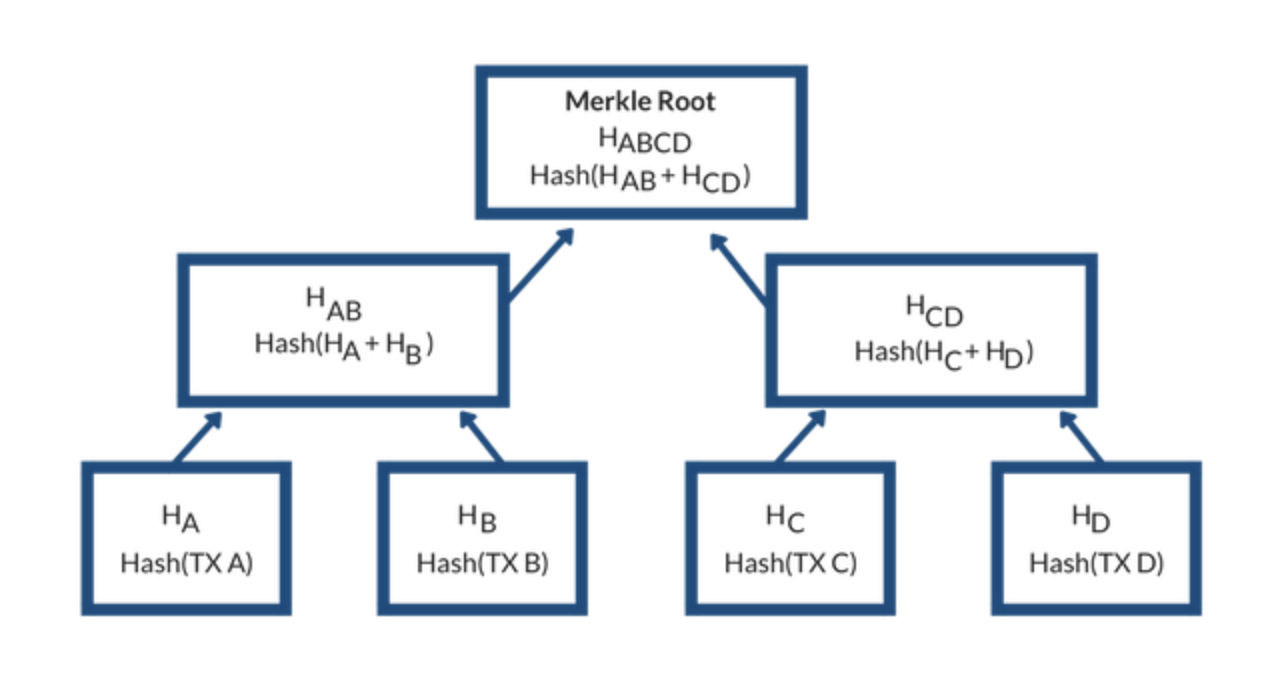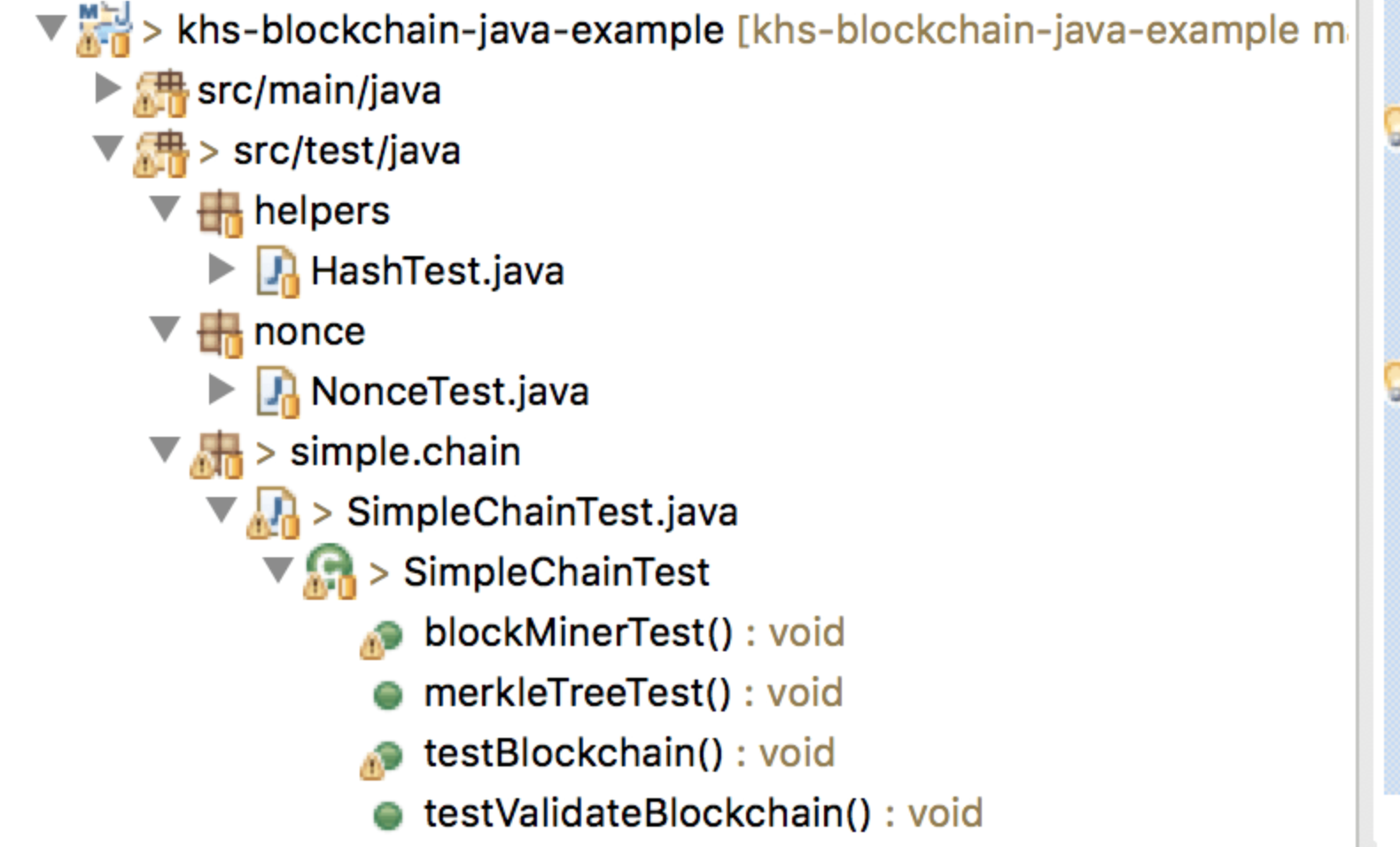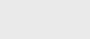2年前 (2022-03-16) |   抢沙发  230

[收起] 文章目录

## 不变性

``````...
public class Block<T extends Tx> {
public long timeStamp;
private int index;
private List<T> transactions = new ArrayList<T>();
private String hash;
private String previousHash;
private String merkleRoot;
private String nonce = "0000";

// caches Transaction SHA256 hashes
public Map<String,T> map = new HashMap<String,T>();
...``````

## 块散列

``````...
public void computeHash() {
Gson parser = new Gson(); // probably should cache this instance
String serializedData = parser.toJson(transactions);
setHash(SHA256.generateHash(timeStamp + index + merkleRoot + serializedData + nonce + previousHash));
}
...``````

## Chain 链

``````...
public class SimpleBlockchain<T extends Tx> {
public static final int BLOCK_SIZE = 10;
public List<Block<T>> chain = new ArrayList<Block<T>>();

public SimpleBlockchain() {
// create genesis block
}

...``````

``````public Block<T> newBlock() {
int count = chain.size();
String previousHash = "root";

if (count > 0)
previousHash = blockChainHash();

Block<T> block = new Block<T>();

block.setTimeStamp(System.currentTimeMillis());
block.setIndex(count);
block.setPreviousHash(previousHash);
return block;
}``````

``````public void addAndValidateBlock(Block<T> block) {

// compare previous block hash, add if valid
Block<T> current = block;
for (int i = chain.size() - 1; i >= 0; i--) {
Block<T> b = chain.get(i);
if (b.getHash().equals(current.getPreviousHash())) {
current = b;
} else {

throw new RuntimeException("Block Invalid");
}

}

}``````

``````public boolean validate() {

String previousHash = null;
for (Block<T> block : chain) {
String currentHash = block.getHash();
if (!currentHash.equals(previousHash)) {
return false;
}

previousHash = currentHash;

}

return true;

}``````

## Merkle树``````public List<String> merkleTree() {
ArrayList<String> tree = new ArrayList<>();
// Start by adding all the hashes of the transactions as leaves of the
// tree.
for (T t : transactions) {
}
int levelOffset = 0; // Offset in the list where the currently processed
// level starts.
// Step through each level, stopping when we reach the root (levelSize
// == 1).
for (int levelSize = transactions.size(); levelSize > 1; levelSize = (levelSize + 1) / 2) {
// For each pair of nodes on that level:
for (int left = 0; left < levelSize; left += 2) {
// The right hand node can be the same as the left hand, in the
// case where we don't have enough
// transactions.
int right = Math.min(left + 1, levelSize - 1);
String tleft = tree.get(levelOffset + left);
String tright = tree.get(levelOffset + right);
}
// Move to the next level.
levelOffset += levelSize;
}
return tree;
}``````

``````@Test
public void merkleTreeTest() {

SimpleBlockchain<Transaction> chain1 = new SimpleBlockchain<Transaction>();

// get a block in chain

System.out.println("Merkle Hash tree :" + block.merkleTree());

// get a transaction from block
Transaction tx = block.getTransactions().get(0);

// see if block transactions are valid, they should be
block.transasctionsValid();
assertTrue(block.transasctionsValid());

// mutate the data of a transaction
tx.setValue("Z");

// block should no longer be valid, blocks MerkleRoot does not match computed merkle tree of transactions
assertFalse(block.transasctionsValid());

}``````

## 采矿工作证明

``````private String proofOfWork(Block block) {

String nonceKey = block.getNonce();
long nonce = 0;
boolean nonceFound = false;
String nonceHash = "";

Gson parser = new Gson();
String serializedData = parser.toJson(transactionPool);
String message = block.getTimeStamp() + block.getIndex() + block.getMerkleRoot() + serializedData
+ block.getPreviousHash();

while (!nonceFound) {

nonceHash = SHA256.generateHash(message + nonce);
nonceFound = nonceHash.substring(0, nonceKey.length()).equals(nonceKey);
nonce++;

}

return nonceHash;

}``````

## 单元测试## 最后的想法### 注册